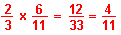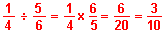Fraction Calculator & Tutorial Multiplication and Division I N S T R U C T I O N S This calculator may look confusing at first and that is why there is a pre-loaded problem for you to look at. The problem is 6/35 multiplied by 10/21. If you click "MULTIPLY" you will see that this equals 60/735 which reduces to 4/49. You can also click the "DIVIDE" button and find that 6/35 divided by 10/21 equals 126/350 which reduces to 9/25. To clear the boxes click the "RESET" button and then you can enter the fractions that you need calculated. When entering negative fraction(s), put a minus sign ONLY on the numerator(s). Fraction Multiplication Compared to fraction addition and subtraction, fraction multiplication is fairly simple. What we do is multiply the numerators and then multiply the denominators. Let's take an example such as 2/3 times 6/11.Fraction Division The easiest way to do fraction division is to invert the terms of the second fraction and then multiply. Here's an example - what is 1/4 divided by 5/6 ?Return To Home Page Copyright © 1999 - 1728 Software Systems